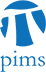## DG-MP-PDE Seminar: Nonlinear singular operators and measure data quasilinear Riccati type equations with nonstandard growth

• Date: 04/06/2010
Lecturer(s):
Phuc Cong Nguyen (Louisiana State University)
Location:

University of British Columbia

Description:

We establish explicit criteria of solvability for the quasilinear Riccati type equation $-\Delta_p u =|\nabla u|^q + \omega$ in a bounded $\mathcal{C}^1$ domain $\Omega\subset\mathbb{R}^n$, $n\geq 2$. Here $\Delta_p$, $p>1$, is the $p$-Laplacian, $q$ is critical $q=p$, or super critical $q>p$, and the datum $\omega$ is a measure. Our existence criteria are given in the form of potential theoretic or geometric (capacitary) estimates that are sharp when $\omega$ is compactly supported in the ground domain $\Omega$. A key in our approach to this problem is capacitary inequalities for certain nonlinear singular operators arising from the $p$-Laplacian.

Schedule:

3:30 - 4:30pm, WMAX 110.